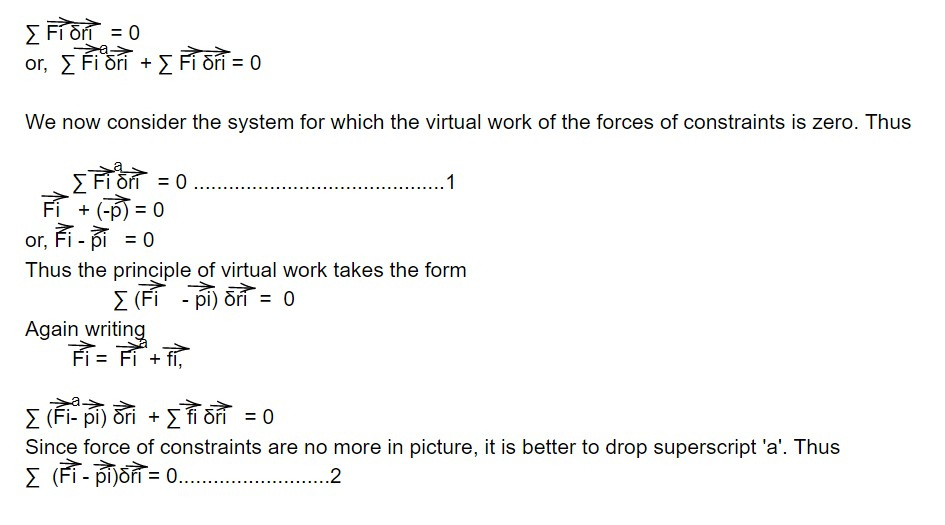# D'Alembert's principle

Consider a system in equilibrium, i.e. total force Fi on every particle is zero. Then work done by this force in a small virtual displacement δr will also vanish i.e., for whole system for N particles.which is called D'Alembert's principle. The key point is that in 2 we got rid of the forces of constraints. To satisfy equation 2, we can't equate the coefficients of δri to zero since δri are not independent of each other and hence it is necessary to transform δri changes into the changes generalized coordinates δqj which are independent of each other.

This note is taken from Classical mechanic, Physics, Nepal.